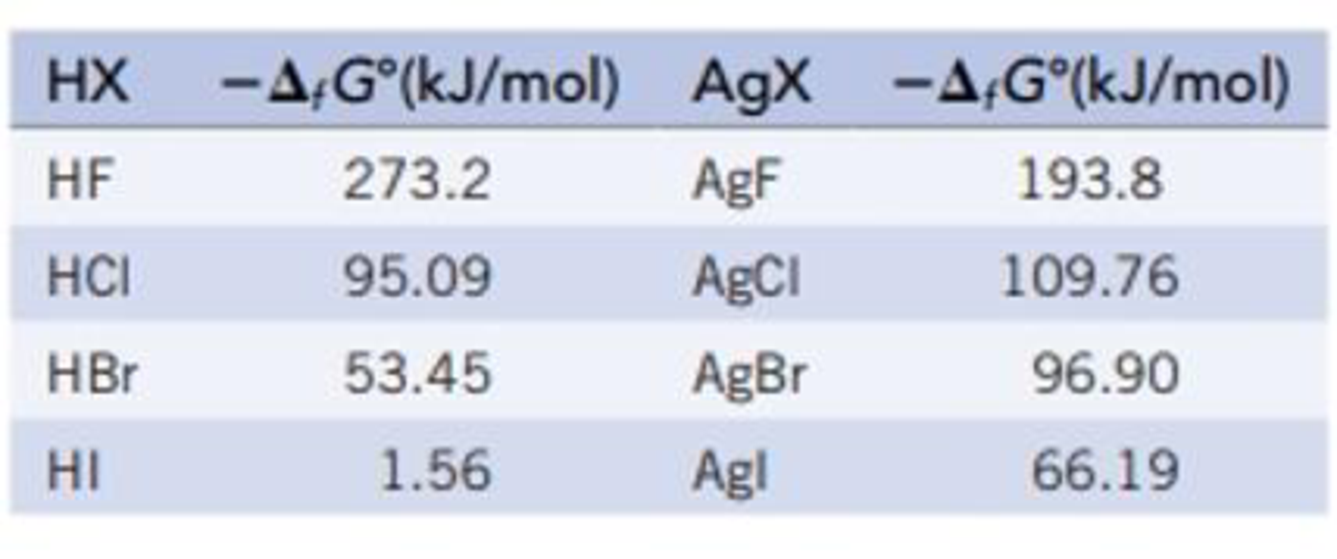Chapter 21, Problem 114GQ

Chapter
Section
Textbook Problem

Metals generally react with hydrogen halides to give the metal halide and hydrogen. Determine whether this is true for silver by calculating ΔrG° for the reaction with each of the hydrogen halides.Ag(s) + HX(g) → AgX(s) + ½ H2(g)The required free energies of formation are:Interpretation Introduction

Interpretation:

To determine the ΔrG° for the reaction of silver with HX where X is a halogen.

Concept introduction:

The Gibbs free energy or the free energy change is a thermodynamic quantity represented by ΔrG. It can be calculated in a similar manner as entropy and enthalpy.  The expression for the free energy change is:

ΔrG°=nΔfG°(products)nΔfG°(reactants)

It is related to entropy and entropy by the following expression,

ΔrG=ΔrHTΔrS

Here, ΔrH is the change in enthalpy and ΔrS is the change in entropy.

Explanation

The ΔrG for the reaction of silver with hydrogen halide is calculated below.

Given:

Refer to Appendix L for the values of standard free energies.

For X=F,

The given reaction is,

Ag(s)+HF(g)AgF(s)+12H2(g)

The ΔfG° for AgF(s) is 193.8 kJ/mol.

The ΔfG° for HF(g) is 273.2 kJ/mol.

The ΔfG° for H2(g) is 0 kJ/mol.

The ΔfG° for Ag(s) is 0 kJ/mol.

The expression for free energy change is,

ΔrG°=nΔfG°(products)nΔfG°(reactants)=[[(1 mol AgF(s)/mol-rxn)ΔfG°[AgF(s)]+(0.5 mol H2(g)/mol-rxn)ΔfG°[H2(g)]][(1 mol Ag(s)/mol-rxn)ΔfG°[Ag(s)]+(1 mol HF(g)/mol-rxn)ΔfG°[HF(g)]] ]

Substitute the values,

ΔrG°=[[(1 mol AgF(s)/mol-rxn)(193.8 kJ/mol)+(0.5 mol H2(g)/mol-rxn)(0 kJ/mol)][(1 mol Ag(s)/mol-rxn)(0 kJ/mol)+(1 mol HF(g)/mol-rxn)(273.2 kJ/mol)] ] =+79.4 kJ/mol-rxn

For X=Cl,

The given reaction is,

Ag(s)+HCl(g)AgCl(s)+12H2(g)

The ΔfG° for AgCl(s) is 109.76 kJ/mol.

The ΔfG° for HCl(g) is 95.09 kJ/mol.

The ΔfG° for H2(g) is 0 kJ/mol.

The ΔfG° for Ag(s) is 0 kJ/mol.

The expression for free energy change is,

ΔrG°=nΔfG°(products)nΔfG°(reactants)=[[(1 mol AgCl(s)/mol-rxn)ΔfG°[AgCl(s)]+(0.5 mol H2(g)/mol-rxn)ΔfG°[H2(g)]][(1 mol Ag(s)/mol-rxn)ΔfG°[Ag(s)]+(1 mol HCl(g)/mol-rxn)ΔfG°[HCl(g)]] ]

Substitute the values,

ΔrG°=[[(1 mol AgCl(s)/mol-rxn)(109.76 kJ/mol)+(0.5 mol H2(g)/mol-rxn)(0 kJ/mol)][(1 mol Ag(s)/mol-rxn)(0 kJ/mol)+(1 mol HCl(g)/mol-rxn)(95.09 kJ/mol)] ] =14.67 kJ/mol-rxn

For X=Br,

The given reaction is,

Ag(s)+HBr(g)AgBr(s)+12H2(g)

The ΔfG° for AgBr(s) is 96

Still sussing out bartleby?

Check out a sample textbook solution.

See a sample solution

The Solution to Your Study Problems

Bartleby provides explanations to thousands of textbook problems written by our experts, many with advanced degrees!

Get Started

Find more solutions based on key concepts